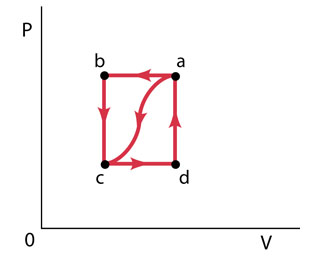Nannie Mack

2021-05-04When a gas is taken from a to c along the curved path in the figure (Figure 1) , the work done by the gas is W = -40 J and the heat added to the gas is Q = -140 J . Along path abc, the work done by the gas is W = -50 J . (That is, 50 J of work is done on the gas.)
I keep on missing Part D. The answer for part D is not -150,150,-155,108,105( was close but it said not quite check calculations)
Part A
What is Q for path abc?
Express your answer to two significant figures and include the appropriate units.
Part B
f Pc=1/2Pb, what is W for path cda?
Express your answer to two significant figures and include the appropriate units.
Part C
What is Q for path cda?
Express your answer to two significant figures and include the appropriate units.
Part D
What is Ua?Uc?
Express your answer to two significant figures and include the appropriate units.
Part E
If Ud?Uc=42J, what is Q for path da?
Express your answer to two significant figures and include the appropriate units.Anonym

Part D:
For the variation of the internal energy in the path cda we have that
$\mathrm{△}{U}_{ca}=\mathrm{△}{U}_{cd}+\mathrm{△}{U}_{da}$
$\mathrm{△}{U}_{ca}=\left({Q}_{cd}-{W}_{cd}\right)+\left({Q}_{da}-{W}_{da}\right)$
the path da is a constant volume, so the work in this path is zero
$\mathrm{△}{U}_{ca}=\left({Q}_{cd}-{W}_{cd}\right)+\left({Q}_{da}-0\right)$
$\mathrm{△}{U}_{ca}={Q}_{cd}+{Q}_{da}-{W}_{cd}$
$\mathrm{△}{U}_{ca}={Q}_{ca}-{W}_{cd}$
Here we have, form the parts B and C, the ${Q}_{ca}=130J$ and the , so
$\mathrm{△}{U}_{ca}=105J$
An Answer that you already said is incorrect. But there is a "trick", the significant figures. You need an answer with two significant figures but that has three. So, rounding, the answer is
$\mathrm{△}{U}_{ca}=110J$
Part E:
Since the path dc is at constant volume
$\mathrm{△}{U}_{cd}={Q}_{cd}$
And like we have ${Q}_{cda}$
${Q}_{cda}={Q}_{cd}+{Q}_{da}$
${Q}_{da}={Q}_{cda}-{Q}_{cd}$
${Q}_{da}=130J-42J$
${Q}_{da}=88J$

Do you have a similar question?